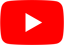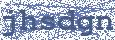Let It Rise

Let It Rise Lyrics

Big Daddy Weave - "Let It Rise"

Let the glory of the Lord
Rise among us
Let the glory of the Lord
Rise among us
Let the praises of the King
Rise among us
Let it rise

Let the songs of the Lord
Rise among us
Let the songs of the Lord
Rise among us
Let the joy of the King
Rise among us
Let it rise

Ohh, ohh ohh
Let it rise

Let the glory of the Lord
Rise among us
Let the glory of the Lord
Rise among us
Let the praises of the King
Rise among us
Let it rise

And, let the songs of the Lord
Rise among us
Let the songs of the Lord
Rise among us
And, let the joy of the King
Rise among us
Let it rise

Ohh, ohh ohh
Let it rise
Ohh, ohh ohh
Let it rise
Ohh, ohh ohh
(5X)Let it rise

Let the glory of the Lord
Rise among us
Let the glory of the Lord
Rise among us
And, let the praises of the King
Rise among us
Let it rise

And, let the songs of the Lord
Rise among us
Let the songs of the Lord
Rise among us
And, let the joy of the King
Rise among us
Let it rise

Ohh, ohh ohh
Let it rise
Ohh, ohh ohh
Let it rise
Ohh, ohh ohh
Let it rise
Ohh, ohh ohh
(8X)Let it riseHottest Lyrics with Videos